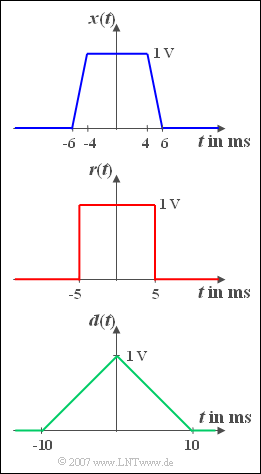# Exercise 3.4Z: Trapezoid, Rectangle and TriangleTrapezoidal pulse and its limiting cases  "Rectangle"  and  "Triangle"

Three different pulse shapes are considered.  The pulse  ${x(t)}$  is trapezoidal.  For  $| t | < t_1 = 4 \,\text{ms}$ the time course is constant equal to  ${A} = 1\, \text{V}$.  Afterwards,  ${x(t)}$  drops linearly to the value zero until the time  $t_2 = 6\, \text{ms}$.

The spectral function of the trapezoidal pulse is

$$X( f ) = A \cdot \Delta t \cdot {\mathop{\rm si}\nolimits}( {{\rm \pi} \cdot \Delta t \cdot f} ) \cdot \hspace{0.1cm}{\mathop{\rm si}\nolimits}( {{\rm \pi}\cdot \Delta t \cdot r_t \cdot f} ).$$

with the two derived system quantities, namely

$$\Delta t = t_1 + t_2,$$
• and the so-called roll-off factor (in the time domain):
$$r_t = \frac{t_2 - t_1 }{t_2 + t_1 }.$$

Furthermore, the rectangular pulse  ${r(t)}$  and the triangular pulse  ${d(t)}$  are also shown in the graph, both of which can be interpreted as limiting cases of the trapezoidal pulse  ${x(t)}$.

Hints:

• This exercise belongs to the chapter  Fourier Transform Theorems.
• You can check your results using the two interactive applets
Pulses and Spectra,
Frequency & Impulse Responses.

### Questions

1

What is the equivalent pulse duration and the rolloff factor of  ${x(t)}$?

 $\Delta t \ = \$  $\text{ms}$ $r_t\hspace{0.3cm} = \$

2

Which statements are true regarding the spectral function  ${X(f)}$ ?

 The spectral value at frequency  $f = 0$  is equal to  $20 \,\text{mV/Hz}$. For the phase function the values  $0$  and  $\pi$  $(180^{\circ})$  are possible. ${X(f)}$  only has zeros at all multiples of  $100 \,\text{Hz}$.

3

Which statements are true regarding the spectral function  ${R(f)}$ ?

 The spectral value at frequency  $f = 0$  is equal to  ${X(f = 0)}$. The values  $0$  and  $\pi$  $(180^{\circ})$  are possible for the phase function. ${R(f)}$  only has zeros at all multiples of  $100 \,\text{Hz}$.

4

Which statements are true regarding the spectral function  ${D(f)}$ ?

 The spectral value at frequency  $f = 0$  is equal to  ${X(f = 0)}$. The values  $0$  and  $\pi$  $(180^{\circ})$  are possible for the phase function. ${D(f)}$  only has zeros at all multiples of  $100 \,\text{Hz}$.

### Solution

#### Solution

(1)  The equivalent pulse duration is  $\Delta t = t_1 + t_2 \;\underline{= 10 \,\text{ms}}$  and the rolloff factor is  $r_t = 2/10 \;\underline{= 0.2}$.

(2)  Proposed solutions 2 and 3 are correct:

• The spectral value at  $f = 0$  is  $A \cdot \Delta t = 10 \,\text{mV/Hz}$.
• Since  ${X(f)}$  is real and can assume both positive and negative values, only the two phase values  $0$  und  $\pi$  are possible.
• Zeros exist due to the first si–function at all multiples of  $1/\Delta t = 100\, \text{Hz}$.
• The second si–function leads to zero crossings at intervals of  $1/(r_t \cdot \Delta t) = 500 \,\text{Hz}$.  These coincide exactly with the zeros of the first si–function.

(3)  All proposed solutions are correct:

• With the equivalent pulse duration  $\Delta t = 10 \,\text{ms}$  and the rolloff factor  $r_t = 0$  one obtains:   $R( f ) = A \cdot \Delta t \cdot {\mathop{\rm si}\nolimits} ( {{\rm{\pi }} \cdot \Delta t \cdot f} ).$
• It follows that  $R( f = 0) = A \cdot \Delta t = X( f = 0).$

(4)  Proposed solutions 2 and 3 are correct:

• For the triangular pulse, the rolloff factor is  $r_t = 1$.
• The equivalent pulse duration is  $\Delta t = 10 \,\text{ms}$.  It follows that   $D( f ) = A \cdot \Delta t \cdot {\mathop{\rm si}\nolimits} ^2 ( {{\rm{\pi }} \cdot \Delta t \cdot f} )$  and  $D( f = 0) = A \cdot \Delta t = X( f = 0)$.
• Since  ${D(f)}$  cannot become negative, the phase  $[{\rm arc} \; {D(f)}]$  is always zero.  The phase value  $\pi$  $(180°)$  is therefore not possible with the triangular pulse.